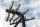# Equation + Pythagorean theorem - examples

1. Find parametersFind parameters of the circle in the plane - coordinates of center and radius: ?
2. Trapezoid MOThe rectangular trapezoid ABCD with right angle at point B, |AC| = 12, |CD| = 8, diagonals are perpendicular to each other. Calculate the perimeter and area of ​​the trapezoid.
3. GardenArea of square garden is 6/4 of triangle garden with sides 56 m, 35 m and 35 m. How many meters of fencing need to fence a square garden?
4. Right ΔRight triangle has length of one leg 28 cm and length of the hypotenuse 53 cm. Calculate the height of the triangle.
5. Short cutImagine that you are going to the friend. That path has a length 330 meters. Then turn left and go another 2000 meters and you are at a friend's. The question is how much the journey will be shorter if you go direct across the field?
6. RiverFrom the observatory 14 m high and 32 m from the river bank, river width appears in the visual angle φ = 20°. Calculate width of the river.
7. Proof PTCan you easy prove Pythagoras theorem using Euclidean theorems? If so, do it.
8. MO SK/CZ Z9–I–3John had the ball that rolled into the pool and it swam in the water. Its highest point was 2 cm above the surface. Diameter of circle that marked the water level on the surface of the ball was 8 cm. Determine the diameter of John ball.
9. Spherical capFrom the sphere of radius 18 was truncated spherical cap. Its height is 12. What part of the volume is spherical cap from whole sphere?
10. R triangleCalculate the area of a right triangle whose longer leg is 6 dm shorter than the hypotenuse and 3 dm longer than the shorter leg.
11. MediansCalculate the sides of a right triangle if the length of the medians to the legs are ta = 21 cm and tb=12 cm.
12. Rectangle SSPerimeter of a rectangle is 268 cm and its diagonal is 99.3 cm. Determine the dimensions of the rectangle.
13. ISO triangleCalculate the area of an isosceles triangle KLM if the length of its sides are in the ratio k:l:m = 4:4:3 and has perimeter 377 mm.
14. Leg and heightSolve right triangle with height v = 9.6 m and shorter cathetus b = 17.3 m.
15. CircleCircle touch two parallel lines p and q; and its center lies on a line a, which is secant of lines p and q. Write the equation of circle and determine the coordinates of the center and radius. p: x-10 = 0 q: -x-19 = 0 a: 9x-4y+5 = 0
16. Trapezoidtrapezoid ABCD a = 35 m, b=28 m c = 11 m and d = 14 m. How to calculate its area?
17. Rectangle diagonalsIt is given rectangle with area 24 cm2 a circumference 20 cm. The length of one side is 2 cm larger than length of second side. Calculate the length of the diagonal. Length and width are yet expressed in natural numbers.
18. Nice prismCalculate the surface of the cuboid if the sum of its edges is a + b + c = 19 cm and the body diagonal size u = 13 cm.
19. ColumnPerpendicular pole high 8 m tall broke and its toe fell 2.7 m from the bottom of the pole. At what height above the ground pole broke?
20. TriangleCalculate the sides of the triangle if its area S = 630 and the second cathethus is shorter by 17.

Do you have an interesting mathematical example that you can't solve it? Enter it, and we can try to solve it.

To this e-mail address, we will reply solution; solved examples are also published here. Please enter e-mail correctly and check whether you don't have a full mailbox.

Do you have a linear equation or system of equations and looking for its solution? Or do you have quadratic equation? Pythagorean theorem is the base for the right triangle calculator.